# Maths Solutions for Ncert Class 6 Chapter 7 Fractions exercise 7.6

Solutions for Class 6 Ncert Maths chapter 7 Fractions exercise 7.6 are given.

First study the textbook lesson Fractions very well.

Observe the example problems and solutions given in the textbook. Try them well.

Observe the given below solutions and try them in your own method.

Ncert maths solutions for class 6
Fractions

Exercise 7.1

Exercise 7.2

Exercise 7.3

M

# Exercise 7.6 Chapter 7 Fractions class 6 maths Ncert solutions

Chapter – 7      Fractions

Exercise 7.6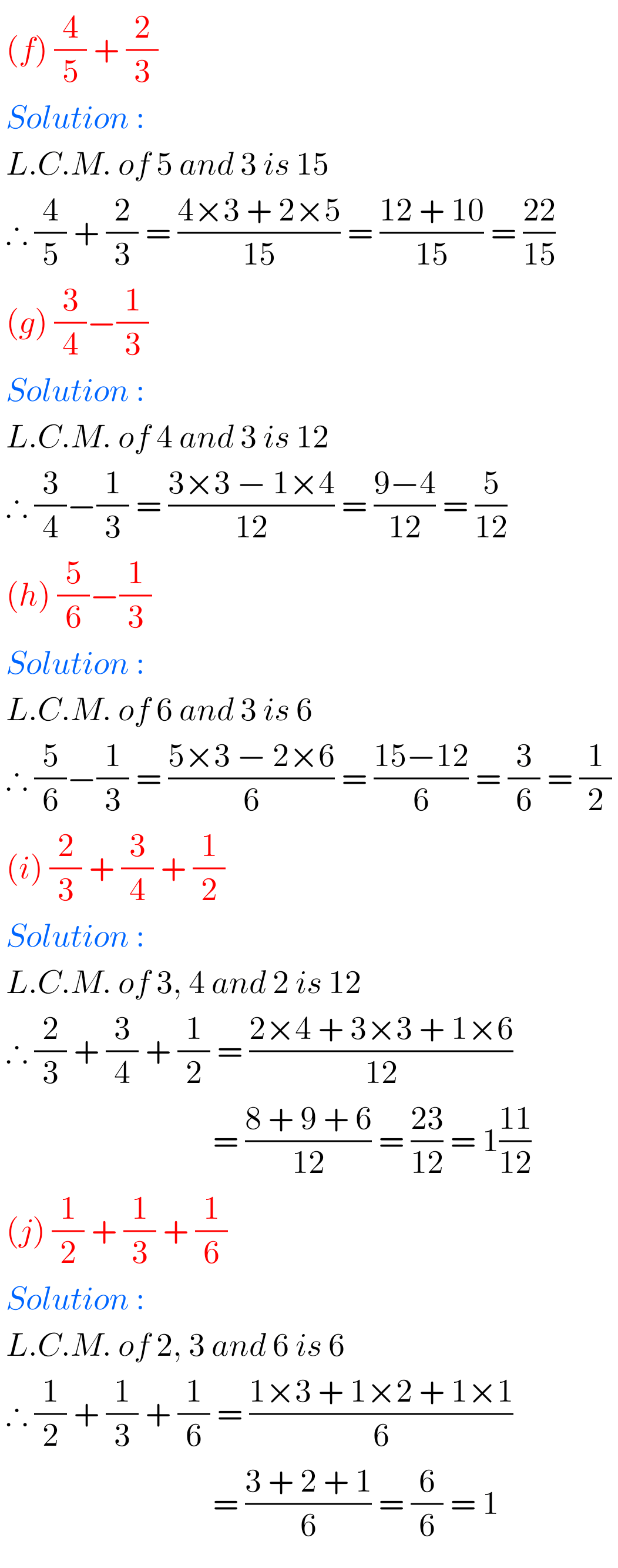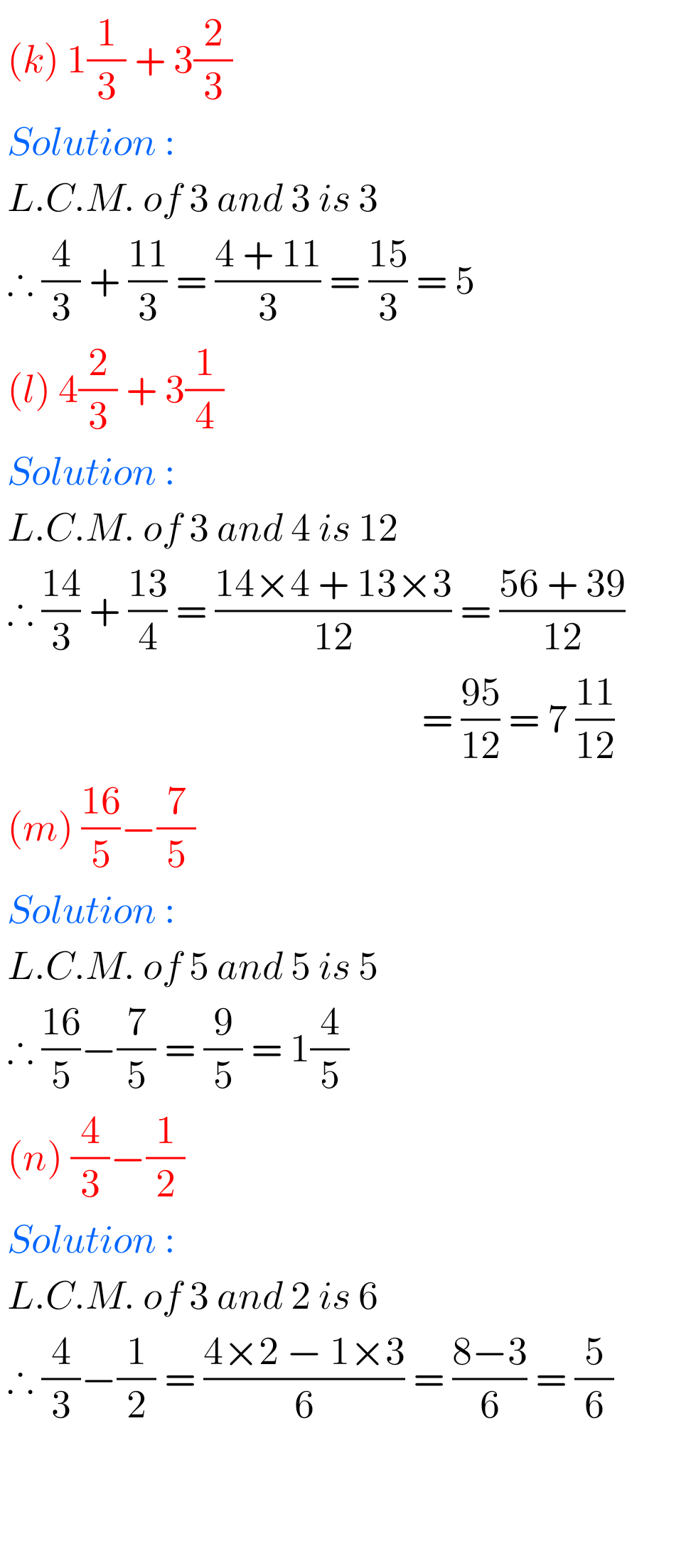## Solutions for maths Ncert class 6 Fractions exercise 7.6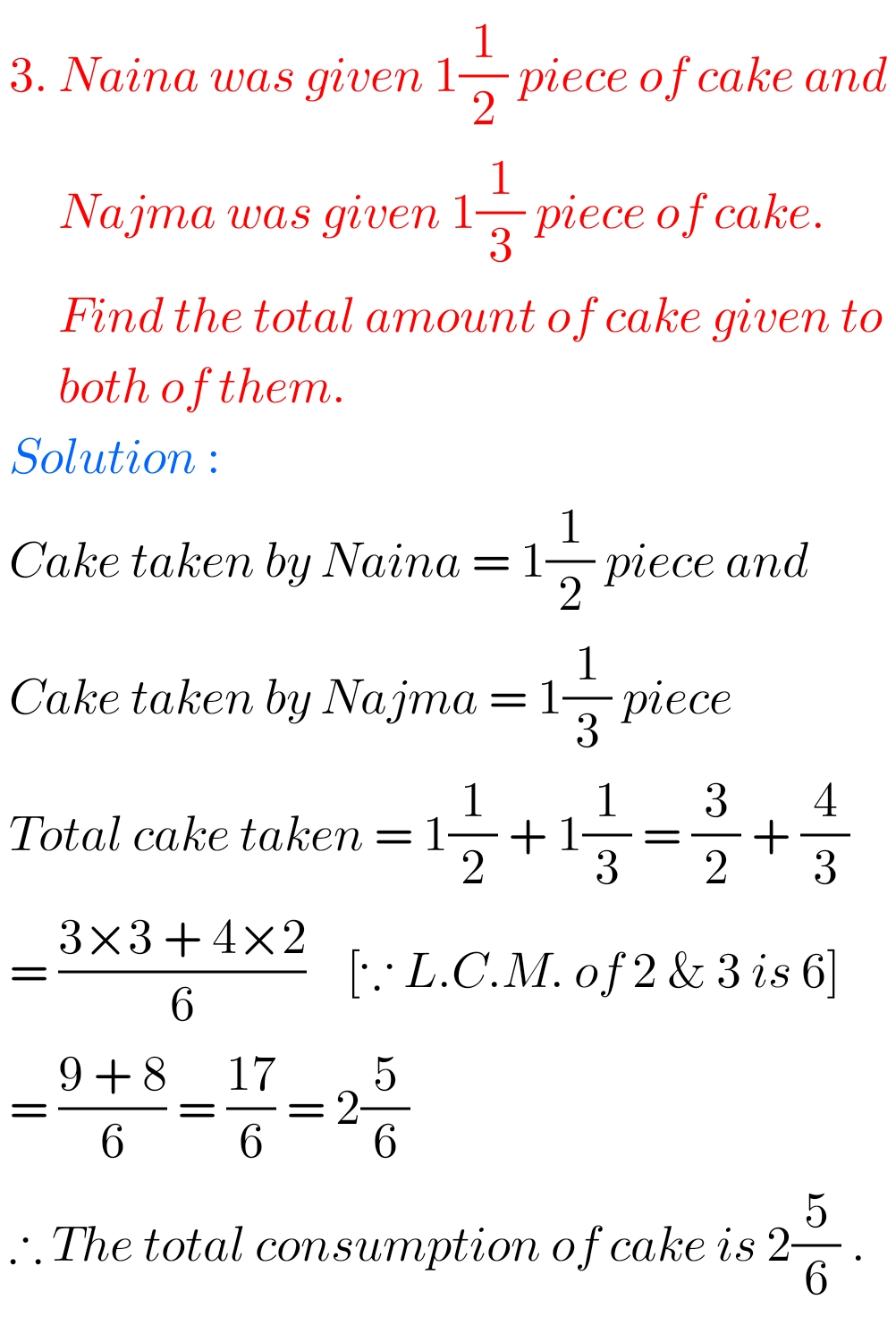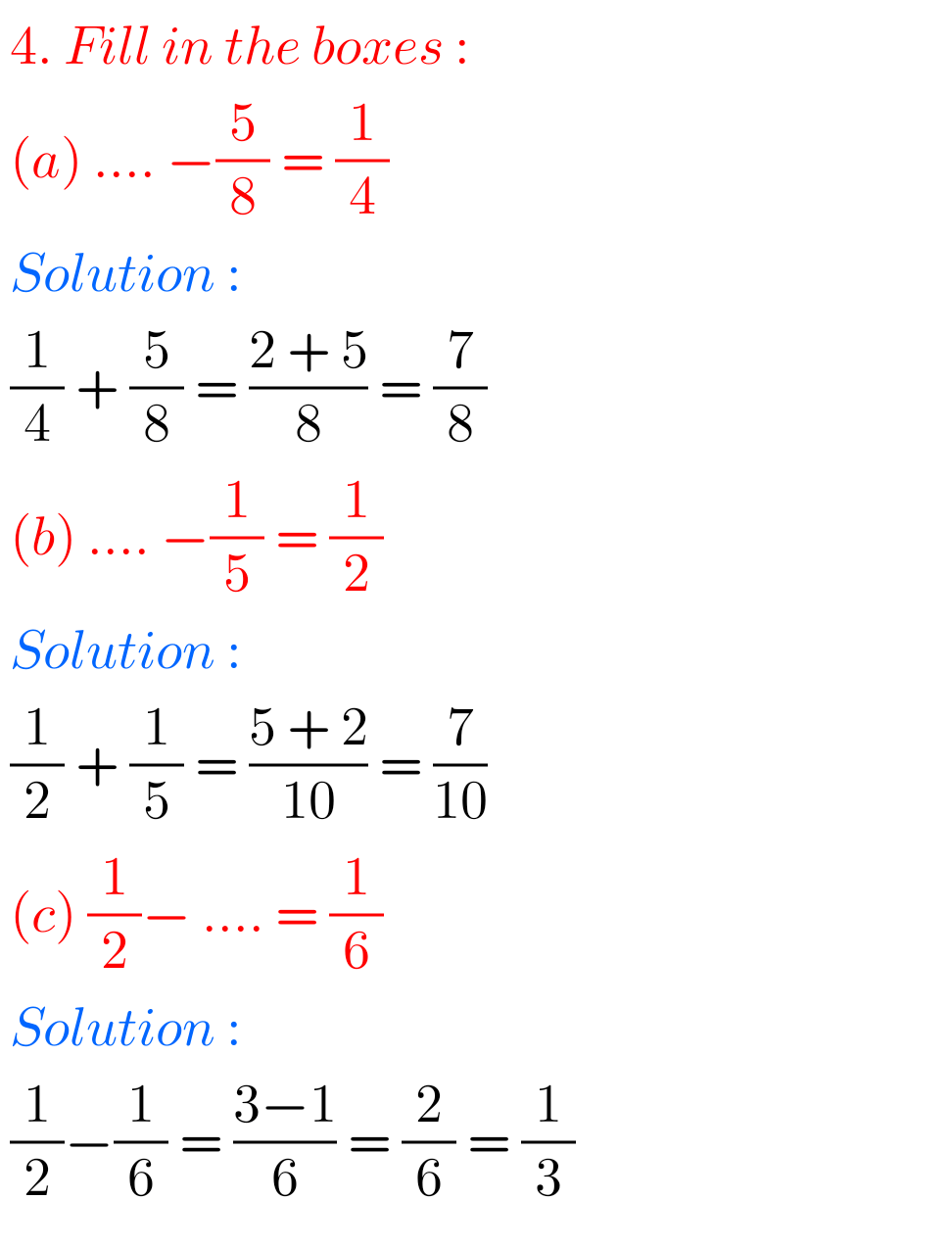F

M

## Chapter 7 Class 6th Fractions Exercise 7.6 solutions Ncert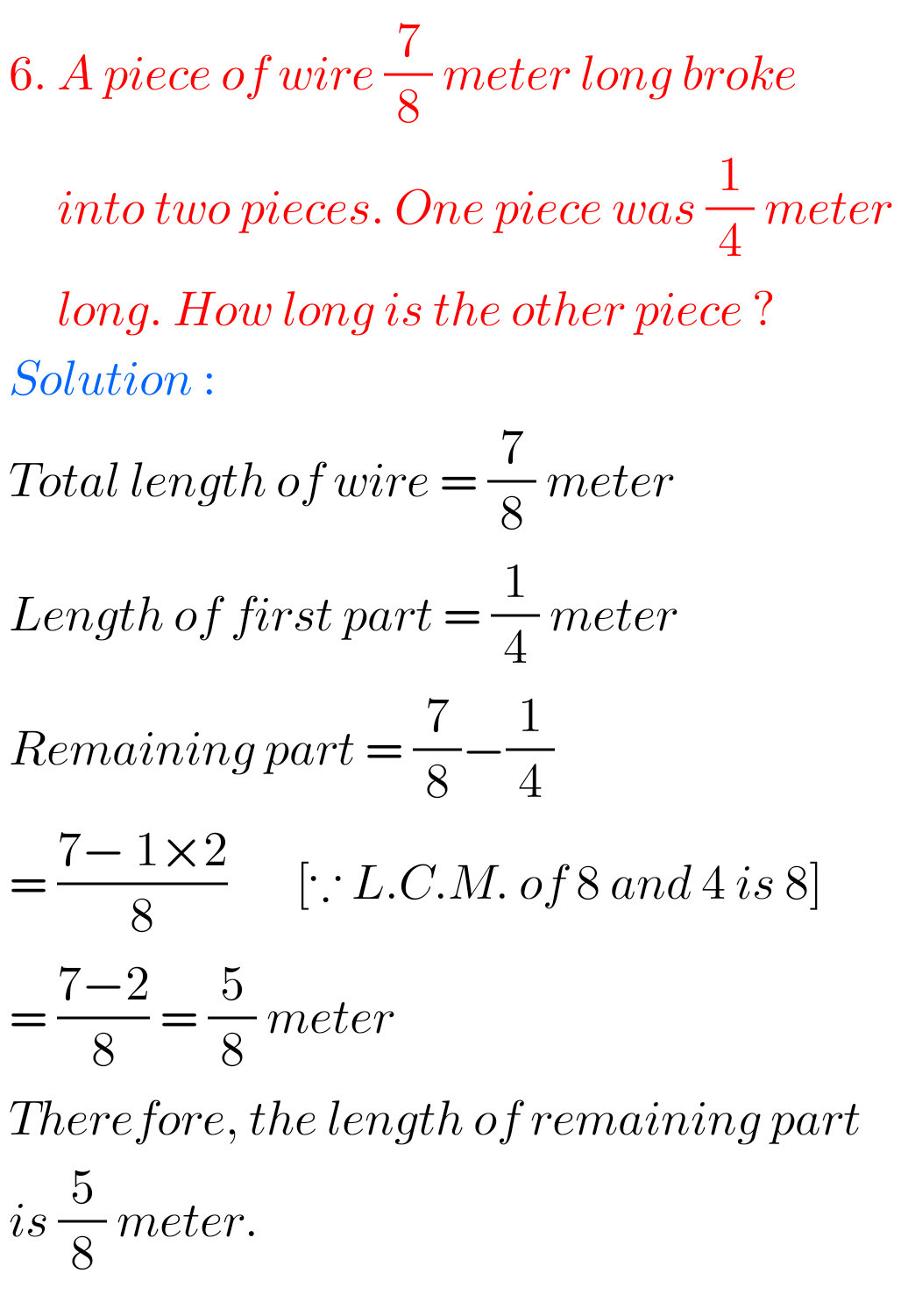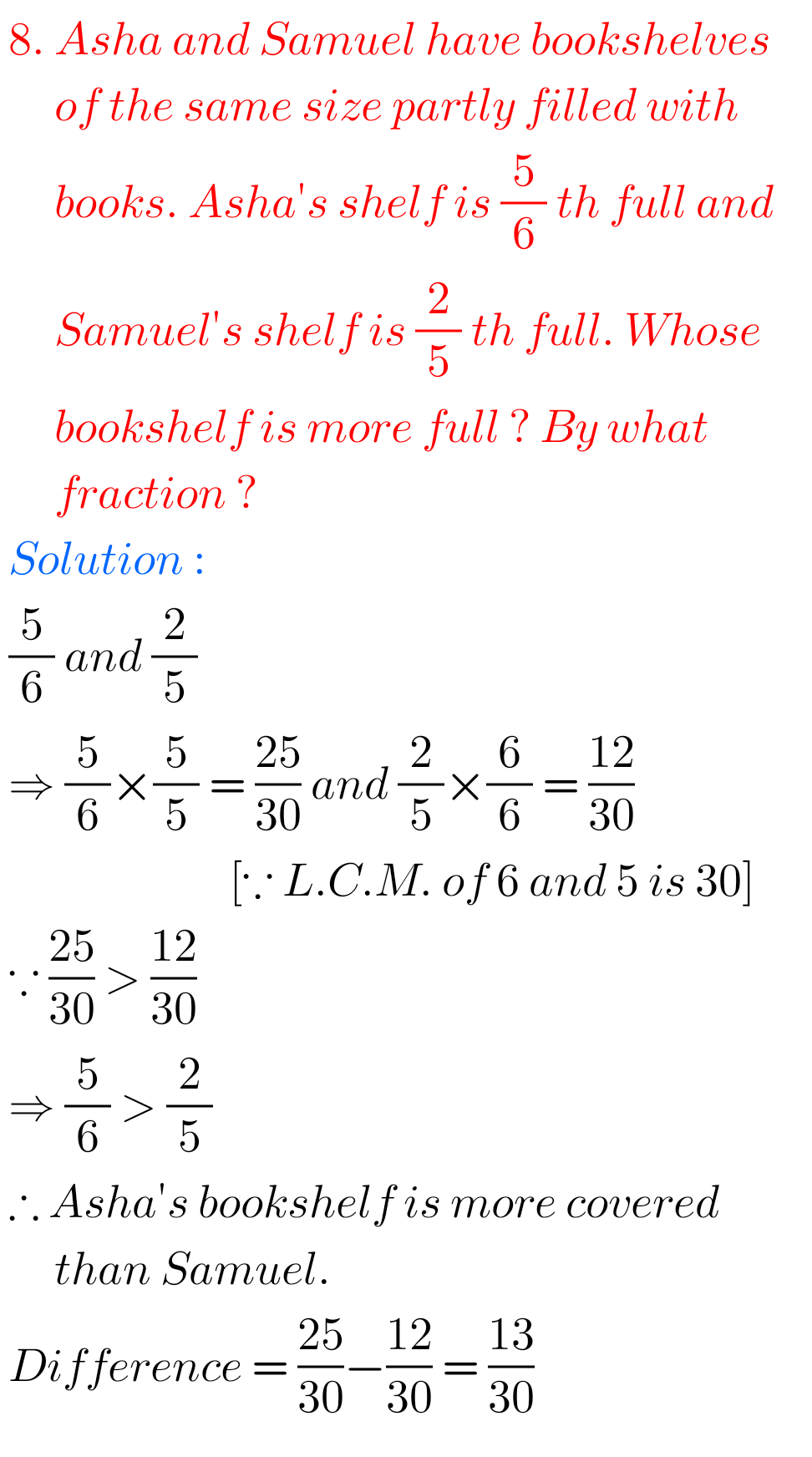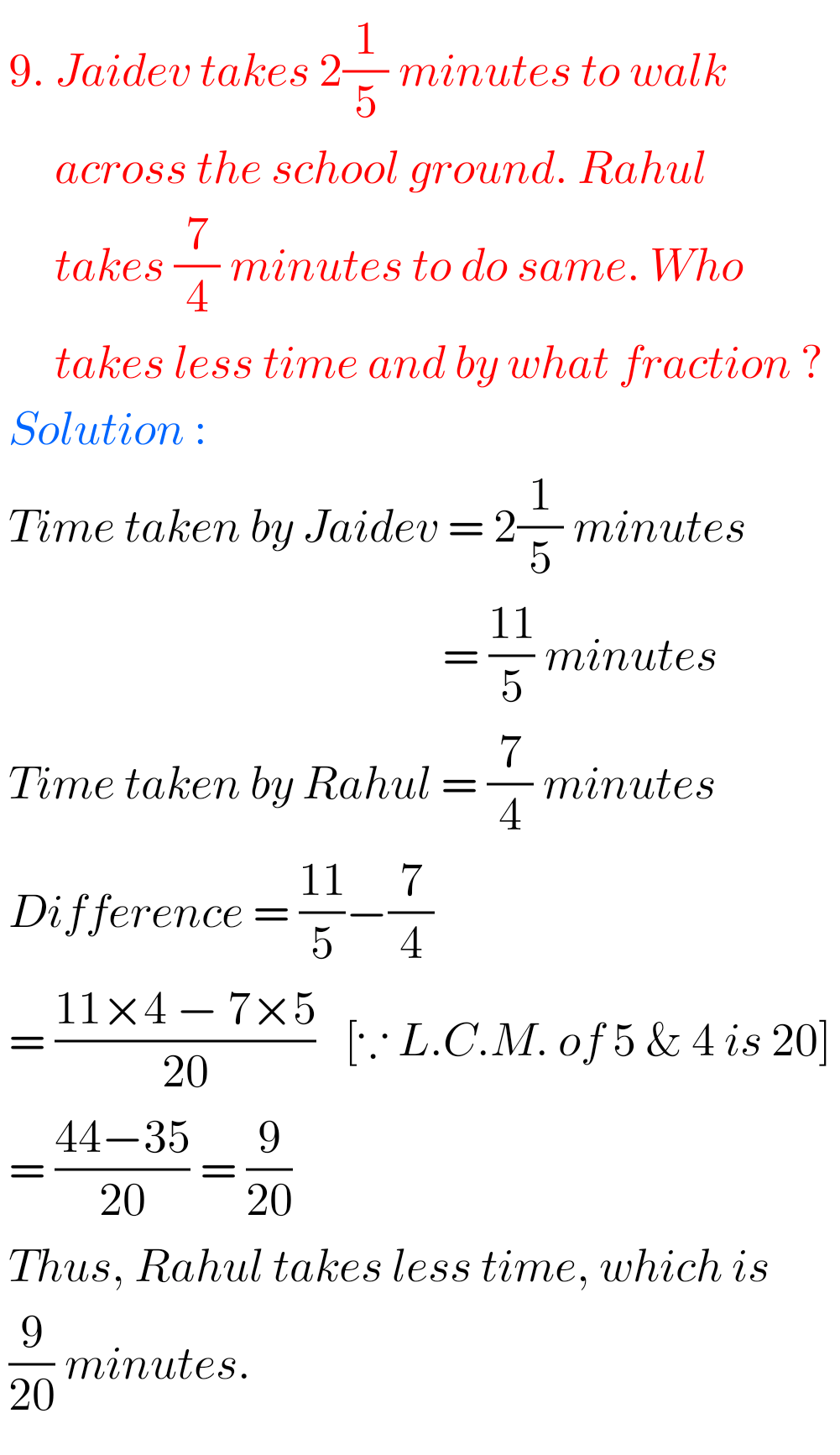Note : Observe the solutions and try them in your own method.